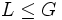# Baer norm is hereditarily permutable

This article gives the statement, and possibly proof, of the fact that for any group, the subgroup obtained by applying a given subgroup-defining function (i.e., Baer norm) always satisfies a particular subgroup property (i.e., hereditarily permutable subgroup)}
View subgroup property satisfactions for subgroup-defining functions$|$ View subgroup property dissatisfactions for subgroup-defining functions

## Statement

### Verbal statement

The Baer norm of any group is a hereditarily permutable subgroup: every subgroup of it is permutable in the whole group.

### Statement with symbols

Suppose$G$ is a group and$B$ is the Baer norm of$G$:$B$ is the intersection of Normalizer (?)s of all the subgroups of$G$. Then,$B$ is a hereditarily permutable subgroup. In other words, if$A$ is a subgroup of$B$, and$L$ is a subgroup of$G$, then$A$ and$L$ permute:$AL = LA$.

## Definitions used

### Baer norm

Further information: Baer norm

The Baer norm of a group is defined as the intersection of normalizers of all its subgroups. In symbols, for a group$G$, the Baer norm$B(G)$ is given by:$B(G) = \bigcap_{H \le G} N_G(H)$.

### Hereditarily permutable subgroup

Further information: Hereditarily permutable subgroup

A subgroup$H \le G$ is termed hereditarily permutable if for every subgroup$K \le H$ and every subgroup$L \le G$,$KL = LK$, i.e.,$K$ and$L$ are permuting subgroups.

## Related facts

• Baer norm not is hereditarily normal: The Baer norm of a group need not be hereditarily normal: it may have subgroups that are not normal in the whole group.
• Baer norm is Dedekind: Every subgroup of the Baer norm is normal inside the Baer norm (though, as pointed above, it need not be normal in the whole group).

## Proof

Given: A group$G$ with Baer norm$B$. A subgroup$A \le B$ and a subgroup$L \le G$.

To prove:$AL = LA$.

Proof:

1.$A \le N_G(L)$: Since$B$ is the intersection of normalizers of all subgroups of$G$,$B \le N_G(L)$. In particular, since$A \le B$, we have$A \le N_G(L)$.
2.$AL = LA$: Since$A \le N_G(L)$, we have$aL = La$ for all$a \in A$. Thus,$AL$, which is defined as the union of the$aL, a \in A$, must equal$LA$, which is defined as the union of the$La, a \in A$.